# voltmeter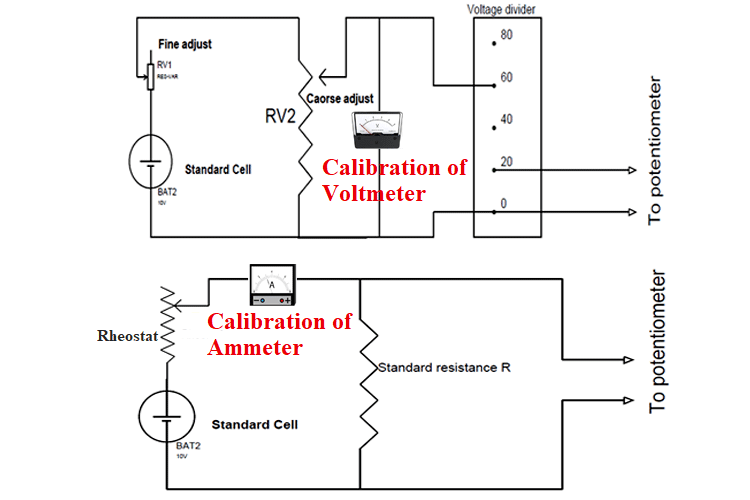Calibration of Ammeter, Voltmeter, and Wattmeter using PotentiometerWe know that voltage, current, and power are measured in volts, amps and, watts and voltmeter, ammeter, and wattmeter are used…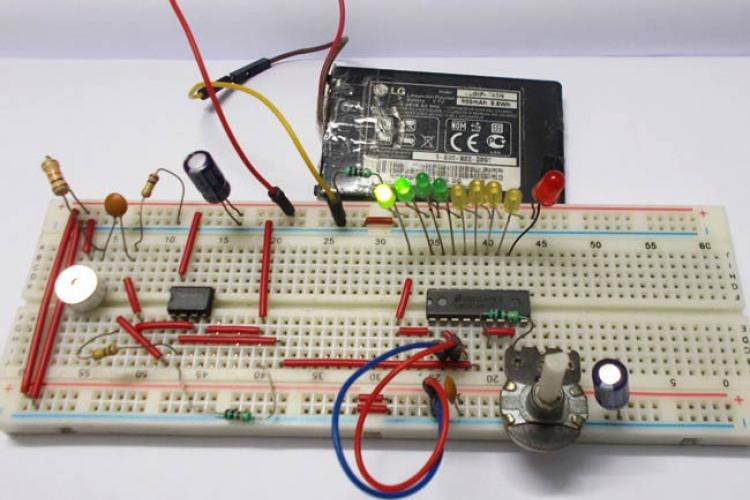LED VU Meter using LM3914 and LM358We can consider the Volume Meter as an Equalizer, which is present in the Music systems. In which we can see the dancing of…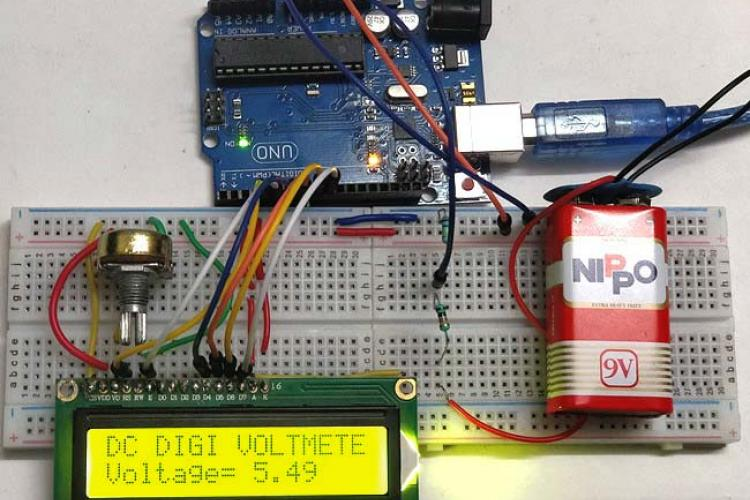Simple Arduino Digital VoltmeterWith a simple knowledge of Arduino and Voltage Divider Circuit, we can turn the Arduino into Digital Voltmeter and can measure…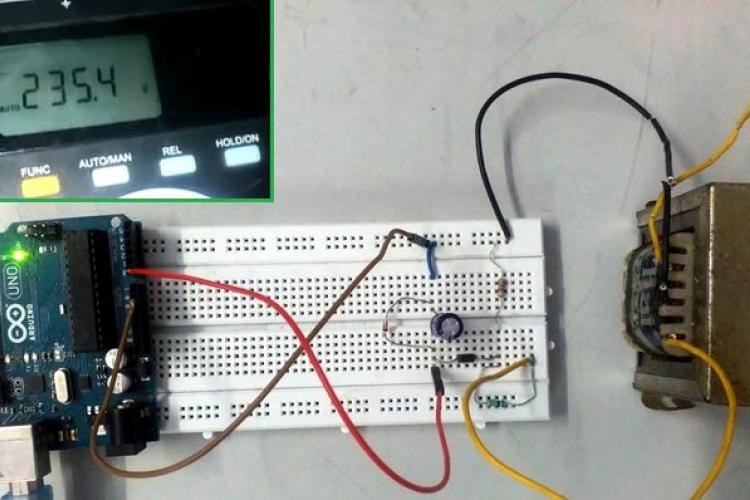AC Voltmeter using ArduinoIn this project, we are going to make an AC Voltage Measuring Device using Arduino, which will measure the voltage of…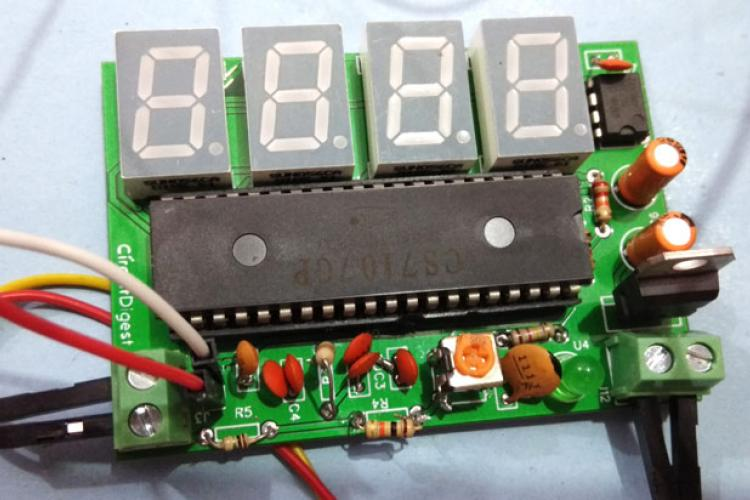Simple Digital Voltmeter Circuit with PCB using ICL7107In this project we are going to build a Digital Voltmeter without using any microcontroller. Here we are using a very popular…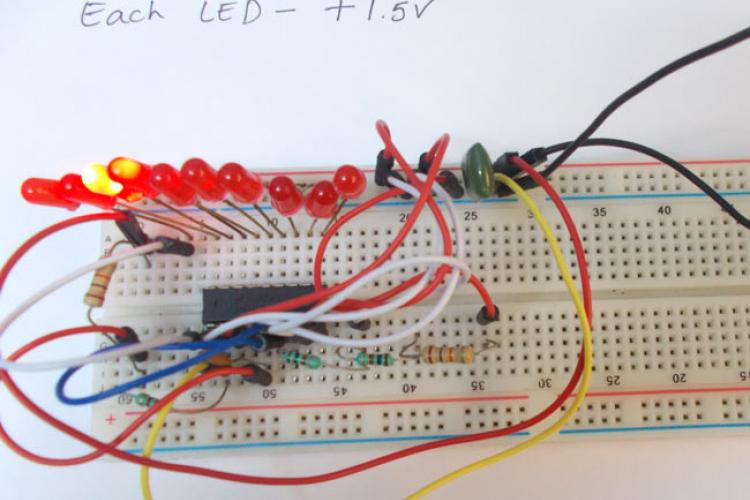LM3914 Voltmeter CircuitHere we are going to design a simple voltmeter. It’s simple because it includes only one IC chip - LM3914. LM3914 is a chip…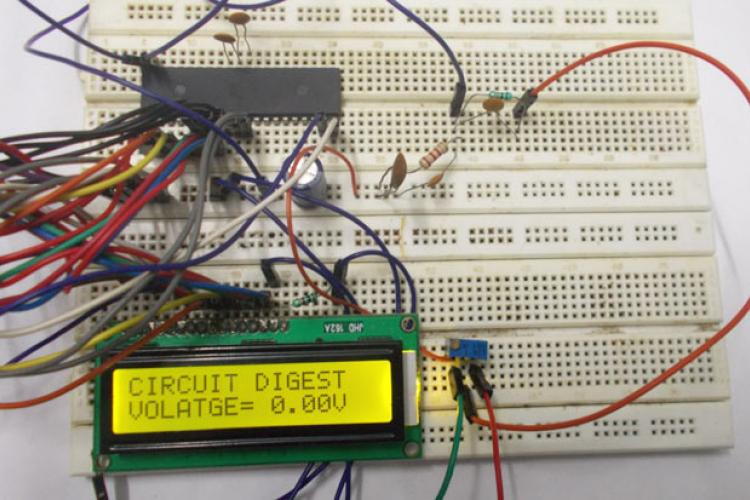0-25V Digital Voltmeter using AVR MicrocontrollerIn this project we are going to design a 25V range digital voltmeter by using ATMEGA32A microcontroller. In ATMEGA, we are…